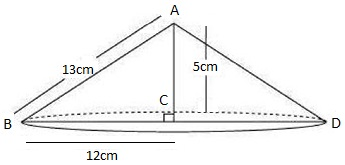Guru

# If the triangle ABC in the Question 7 is revolved about the side 5cm, then find the volume of the solids so obtained. Find also the ratio of the volumes of the two solids obtained in Questions 7 and 8. Q.8

• 0

Yesterday i was doing the question from class 9th ncert book of math of Surface areas and Volumes chapter of exercise 13.7    What is the easiest way for solving it because i was not able to do this question please help me for solving this question no 8 If the triangle ABC in the Question 7 is revolved about the side 5cm, then find the volume of the solids so obtained. Find also the ratio of the volumes of the two solids obtained in Questions 7 and 8

Share

1.A right-angled ΔABC is revolved about its side 5cm, a cone will be formed of radius as 12 cm, height as 5 cm, and slant height as 13 cm.

Volume of cone = (1/3) πr2h; where r is the radius and h be the height of cone

= (1/3)×π×12×12×5

= 240 π

The volume of the cones of formed is 240π cm3.

So, required ratio = (result of question 7) / (result of question 8) = (100π)/(240π) = 5/12 = 5:12.

• 0